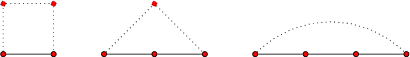## 16 November 2011

### Squaregraphs

On Tuesday, Donald Knuth gave a talk about squaregraphs. I'm summarizing here what I learned, and I'm including the open problems that Knuth mentioned.

Squaregraphs are plannar graphs. When you draw a graph in a plane, each of its cycles partition the plane into two regions, one bounded and one unbounded. When the bounded region is empty (that is, no edge and no vertex is drawn in it), the cycle is called a bounded face; when the unbounded region is empty, the cycle is called an unbounded face. By definition, all bounded faces of a squaregraph are 4-cycles. There is one more condition. A vertex is interior if it does not belong to the unbounded face. All interior vertices must have degree $\ge4$. Here is an example, a gear graph.Knuth told us about many properties that are implied by this definition, usually without proof. I enjoy such talks. First, they give you a hawk's eye view of a subject; second, they make you think about the proofs. Some proofs are easy and some escape you during the talk and even days afterwards, but at least you get an idea of what is easy and what is not. What bothers me is that at no point did I feel the need to invoke the last property, that interior vertices have at least 4 neighbours.

But let's focus on what I did learn, rather than what I did not understand.

While on the bus, before the talk, Rasmus and I looked at the Wikipedia article on squaregraphs, which is very dense, and tried to make sense of it. I think it helped both of us to follow the presentation better. One of the last things Rasmus said was: "Squaregraphs ought to have an inductive definition." And, lo and behold, we found out what that definition is during the talk. Every squaregraph can be built by starting with a 4-cycle and repeatedly adding new faces, subject to the degree constraint: take a simple path of length 1, 2, or 3 that is part of the unbounded face and make a new 4-cycle by adding, respectively, 3, 2, or 1 new edges (drawn in the former unbounded face, that is, towards the outside).Open problem: Is it possible, using this inductive definition, to construct squaregraphs that look like Mona Lisa when viewed from afar?

By the way, a frog can jump from an edge to an opposite edge across a bounded face, obviously. Here is a picture with all possible frog expeditions on an 8-gear.Each expedition, depicted above with a curved green line, is a set of edges that cut the graph into two disconnected parts. We give vertices on one side a bit $0$ and vertices on the other side a bit $1$. If we do this for each expedition then each vertex gets as many bits as there are expeditions. To put some order on these bits we name the expeditions $0$, $1$, $\ldots\,$, $n-1$, so each vertex gets an $n$-bit string. In our example, there are 8 green lines so each vertex will get an 8-bit string.

These bitstrings are interesting because the shortest distance between two vertices equals the Hamming distance between the bitstrings of the vertices. We say that we found an isometric embedding of squaregraphs into an $n$-dimensional hypercube. In $n$ dimensions, squaregraphs look squarish.

Another interesting property of squaregraphs is that they are median graphs. A median graph is one in which all three vertices $x$, $y$, and $z$ have a median vertex $\langle xyz\rangle$ that is on some shortest path between $x$ and $y$, on some shortest path between $y$ and $z$, and on some shortest path between $z$ and $x$. An example is the integer grid, with vertices $(m_x,m_y)$ for all integers $m_x$ and $m_y$, and edges between vertices $m$ and $n$ when $|m_x-n_x|+|m_y-n_y|=1$. The $x$-coordinate of $\langle mnp\rangle$ is the median of the array $[m_x,n_x,p_x]$, hence the name (I guess). It turns out that you can also isometrically embed median graphs in $n$-dimensional hypercubes. That is, you can label vertices with bitstrings such that travelling an edge flips exactly one bit.

A subset of the $n$-dimensional hypercube is a median graph exactly when the selected coordinates are the models of a 2-CNF formula. Knuth said that an open problem would be to find a nice condition to impose on 2-CNF formulas such that their models correspond to squaregraphs.

Notice that on squaregraphs we have a notion of a line (the green equivalence classes above) and also of turning left or right. This means that we can define a knight's move on the bounded faces of a squaregraph. How can we generalize various problems that are usually done on chessboards to squaregraphs? For example, can one define something like rook polynomials for squaregraphs?

Finally, is there a 3D generalization of squaregraphs?

For more information, Knuth recommended a paper by Bandelt, Chepoi, and Eppstein, which is also recommended by Wikipedia. It's quite long, though. Knuth also showed us some nice pictures of squaregraphs on Eppstein's blog. Of this blog he said: "It is one of the best blogs in computer science. I do not read it, for otherwise I would not do anything else."

[Thanks to Rasmus for finding several mistakes in a previous version.]